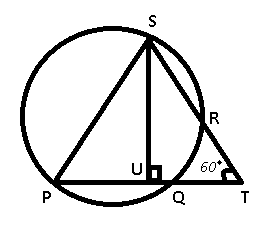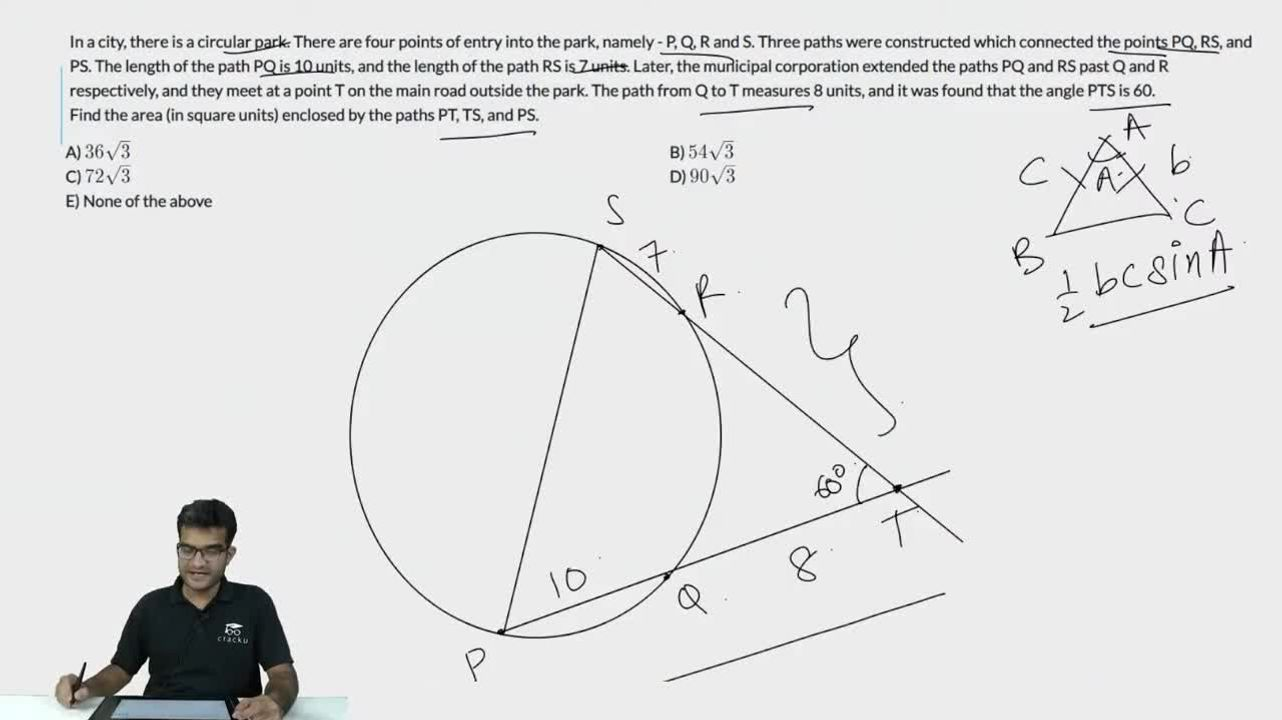Question 80

# In a city, there is a circular park. There are four points of entry into the park, namely - P, Q, R and S. Three paths were constructed which connected the points PQ, RS, and PS. The length of the path PQ is 10 units, and the length of the path RS is 7 units. Later, the municipal corporation extended the paths PQ and RS past Q and R respectively, and they meet at a point T on the main road outside the park. The path from Q to T measures 8 units, and it was found that the angle PTS is 60.Find the area (in square units) enclosed by the paths PT, TS, and PS.

SolutionGiven : PQ = 10 , QT = 8 , RS = 7

Now, TQ*TP=TR*TS (Secant from same external point)

8(PQ+QT)=TR(TR+RS)

8*18=TR(TR+7)

TR=9

TS=9+7=16

$$\therefore ar (\triangle PTS) = \frac{1}{2} \times PT \times TS \times sin60$$

= $$\frac{1}{4} \times (16 \sqrt{3}) \times 18$$

= $$72 \sqrt{3}$$

### View Video Solution